# Basic System Dynamics

Denotes a System Dynamics model containing only compartments, flows and variables

## Urban model (trivial example)

ModelId:
edinburgh1
SimileVersion:
3.1+

This (entirely hypothetical model) aims to demonstrate the potential for developing urban policy simulators. The model contains a number of stocks (industrial capital, population size and landfill), and a number of policy levers that the user can manipulate (road pricing and a rubbish uplift charge). Models like this, expanded and calibrated for particular cities, can be used in a black-box manner, where stakeholders want to see the consequences of different policies.

Equations:

Industrial capital = 1000

Landfill = 1000000
Population size = 500000

Closures = 10
Construction = graph(road_pricing)
Decomposition = 0.2*landfill
Mortality = 9000
Refuse collection = graph(uplift_charge)*population_size
Reproduction = 15000

Age structure = 1
Cars per head = graph(road_pricing)
CO2 = 0.5*emissions+100*industrial_capital+0.5*decomposition Emissions = 1.0e-006*total_cars
Health = temperature*age_structure*graph(pollution)
Note: temperature and age_structure are (at this stage) 0-1 multipliers that cause the effect of pollution to be even worse if either is suboptimal
Pollution = emissions*graph(wind)
Road pricing = 0
Temperature = 1
Total cars = cars_per_head*population_size
Uplift charge = 0
Wind = 2

Model tags:

## Simple degree-days model of temperature influence on development of plants and invertebrates

ModelId:
DegreeDays1
SimileVersion:
4.0

The development rate of plants and invertebrate animals often depends on amount of heat received while the ambient temperature is between minimum and maximum thresholds.

The number of degree-days is a commonly used statistic related to the development of plants and invertebrates. Degree-days, DD, is given by:

DD = ∫ Tt.dt

• T is temperature in degrees Celsius (could be Fahrenheit)
• t is time in days.

degree-days is then oC days or oF days.

Equations:

Compartment DegreeDays : Degree days

Initial value = 0
Rate of change = + dDt on dt
Comments:
Also called temperature sum or less accurately "heat sum".

Flow dDt on dt : Rate of accumation of degree-days (degC)
dDt on dt = max(T_m-T_thresh,0)
Where:
T_m=../Environment/T_m

Variable Sdevelopment : State of development
Sdevelopment = min(DegreeDays/DDreq,1)
Comments:
Value = 0 to 1

Equations in Environment

Variable T_m : Mean daily temperature

Results: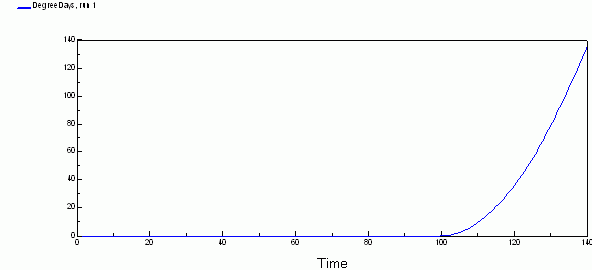## Daisy world

ModelId:
daisyworld1
SimileVersion:
3.1+

This is an implementation of James Lovelock’s DaiyWorld model. This aims to show that even a very simple, artificial system is capable of regulating conditions to suit itself.

We consider a planet on which can grow black amd white daisies. Black daisies heat up the air around them; white daisies cool it down. The proportion of black and white changes as solar output increases, resulting in a reasonably uniform value for global planet temperature.

Model tags:

## Lotka-Volterra two-species competition model

ModelId:
competition1
SimileVersion:
3.1+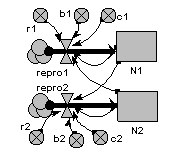This is the classic textbook model of the population dynamics of two competing species.

It is based on a pair of differential equations, each having the same mathematical form:

dX1/dt = r1.X1.(1-b1.X1-c1.X2)
dX2/dt = r2.X2.(1-b2.X2-c2.X1)

Results: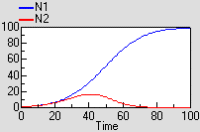## Modelling chemical reactions: CO2 + H2O → Sugar and O2

ModelId:
chemical1
SimileVersion:
5.x

On the face of it, System Dynamics should be a good notation for modelling chemical reactions: chemical reactions are continuous processes involving amounts of substances, and System Dynamics is suitable for modelling continuous processes involvinga mounts of substances.

Equations:

Compartments:

CO2: initial value = 100
H2O: initial value = 100
C6H12O6: initial value = 0
O2: initial value = 0

Flows:
CO2_used = 6*rate
H2O_used = 6*rate
sugar_made = rate
O2_made = 6*rate
from_atmos = 0

Variables:
rate = 0.01*CO2*2*(temperature/10)

Results: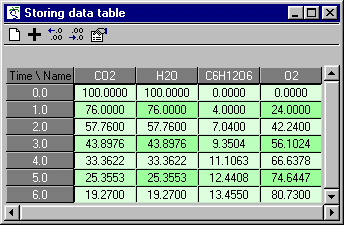Model tags:

## Century model (weekly) (from Cadisch/Rowe)

ModelId:
century_gc_weekly
SimileVersion:
5.x

This implemements the litter and soil organic matter submodels from the Century model (see http://www.nrel.colostate.edu/projects/century5/).

Development history:

• Century2 in Stella by Georg Cadisch;
• Re-implementation of the Stella model in Simile 2.91 by Ed Rowe;
• Converted to Simile version 4.0 by Robert Muetzelfeldt.
•

## Broomgrass model, Zimbabwe

ModelId:
broomgrass1
SimileVersion:
5.x

Co-View is a tool developed by CIFOR (the Centre for International Forestry Research) to help facilitators of natural resource management and stakeholders to articulate and explore a shared vision of the future and to develop strategies to achieve it. The following explanation of the background to the broom grass model is adapted from the CoView (Collaborative Vision Exploration Workbench) User Manual (see Web Links below).

Equations:

Compartments

compartment: broomgrass = 75000

Flows
destroyed = (if burning_internsity==2 then Broom_Grass__in_Machije_Vlei else agriculture+decomposition+element([proportion_of_grass_by__digging_uprooting__or_cutting],1)*total_harvesting/300)
growth = (if frequency_of_burning*burning_internsity>0 and element([proportion_of_grass_by__digging_uprooting__or_cutting],3)>0 then grass_growth*Broom_Grass__in_Machije_Vlei+germination else 0)
total harvesting = (if Broom_Grass__in_Machije_Vlei==0 then 0 elseif harvest then(element([proportion_of_grass_by__digging_uprooting__or_cutting],1)+element([proportion_of_grass_by__digging_uprooting__or_cutting],2))*0.008*number_of_harvesters*quantity_harvested_per_person+element([proportion_of_grass_by__digging_uprooting__or_cutting],3)*0.012*number_of_harvesters*quantity_harvested_per_person else 0)

Variables
agriculture = 2
Percent of Machije converted to agriculture
alternative income sources = (1==0)
availability of transport = (if transport_cost<=10 then 1 else 0)
beauty = processing+bundling_with_tube_or_fibre
bundling with tube or fibre = 1
1 for tube
0 for fibre
burning events = 1
burning internsity = (if season>=6 and season<=10 and frequency_of_burning>0 then 2 else 1)
1 = low internsity burning
2 = high internsity burning
comfort = length
decomposition = 5
expected income after harvesting = number_of_brooms_made*market_price_per_broom-permit_price-transport_cost
expected income before harvesting = market_price_per_broom*2000-permit_price-availability_of_transport
frequency of burning = (if rules_enforcement>0.5 then 0 elseif season>=6 and season<10 then burning_events else 0)
germination = (if soil_moisture==1 then seeds else 0)
grass growth = 1-growth_rate*soil_moisture/K
growth rate = 5
harvest = any([time_of_harvesting]==season)
income = number_of_brooms_sold*market_price_per_broom-permit_price
K = 1000000
length = 1
life of broom = bundling_with_tube_or_fibre*ripe_or_unripe*length*whether_cut__or_dug_or_uprooted
maximum number of broom harvest per day = 100
no_of_permits = 150
number of brooms made = 0.99*total_harvesting
Number of brooms sold = availability_of_transport*number_of_brooms_made
transport]
market price per broom = 3+last(quality_of_broom)*season/(supply_from_other_sources+1)
number of harvesters = (if alternative_income_sources then 0 elseif permit_price0.5 then[5,10,85]else[80,10,10]) quality of broom = beauty*life_of_broom*comfort quantity harvested per person = maximum_number_of_broom__harvest_per_day rainfall = element([rainfall_this_month],season) rainfall this month = [193,185,70,32,7,2,0,0,4,28,91,128] Months of the year when harvesting is permitted ripe or unripe = (if element([time_of_harvesting],1)==6 then 1 else 0) 1 = ripe 0 = unripe season = fmod(time(1),12)+1 seeds = (if element([time_of_harvesting],1)==6 and burning_internsity==1 and season==6 then produce else 0) set harvesting season = [6,7,8,9,10] soil moisture = (if rainfall>100 then 1 else 0) supply from other sources = 1 1 = yes there is supply from elsewhere 0 = there is no availabiility time of harvesting = (if rules_enforcement>0.5 then [set_harvesting_season] elseif number_of_people_not_farming<0 then[set_harvesting_season]-3 else[set_harvesting_season]) time of sale = market_price_per_broom transport_cost = 2 Price in dollars to transport a bundle of brooms

Model tags:

## Simple teaching model of forest nitrogen dynamics

ModelId:
besn1
SimileVersion:
3.1+

### This is a very simple, generic model of nitrogen dynamics in a forest. It was developed as a teaching model, with very simple flow equations and arbitrary parameter values.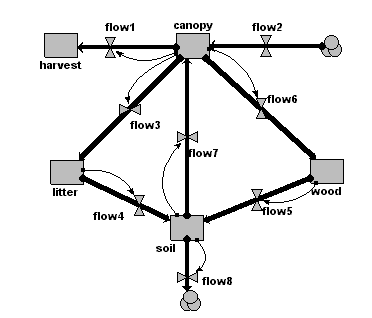Category:
Specific
Equations:

Compartments:
canopy: initial value = 0
harvest: initial value = 0
litter: initial value = 0
wood: initial value = 0
soil: initial value = 0

Flows:
flow1 = 0.9*canopy
flow2 = 50
flow3 = 0.9*canopy
flow4 = 0.9*litter
flow5 = 0.01*wood
flow6 = 0.5*canopy
flow7 = 0.5*soil
flow8 = 0.01*soil

Results: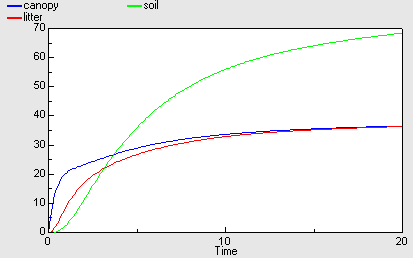Model tags:
Tags: